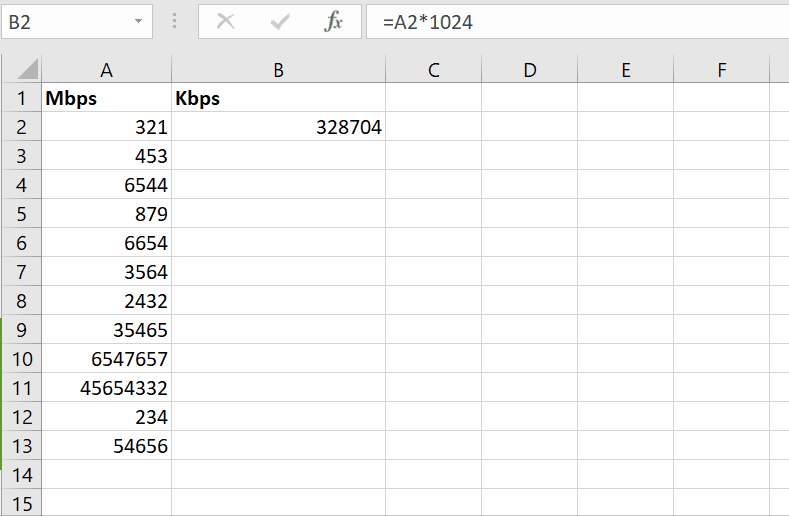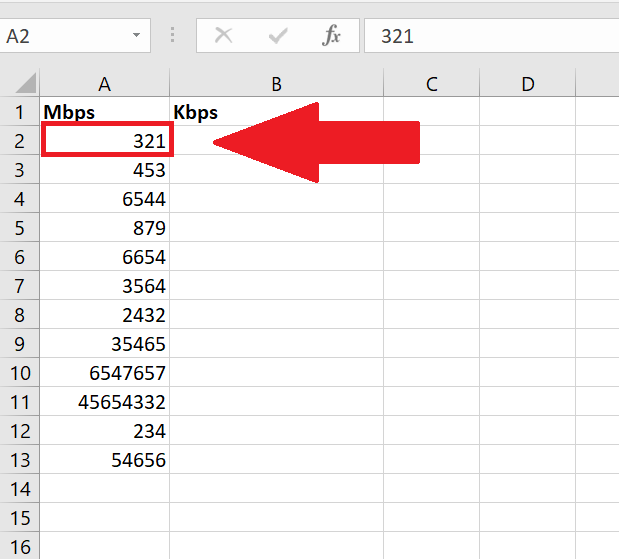# How to convert mbps to kbps

Mbps stands for megabytes per second and Kbps stands for kilobytes per second. As we know, a megabyte is a bigger unit and a kilobyte is a smaller unit. One thing to remember is its conversion factor, which is 1024. 1 mbps = 1024 kbps. We will multiply the mbps value with 1024 to convert it to kbps value.Microsoft Excel is a spreadsheet program designed for daily tasks such as setting up a remittance, conversions, maintaining a contact list, or keeping track of lists to be done. In this tutorial, we’ll learn how to convert different data transfer speed units of a network

### Step 1 – Select the cell in the data range– Select the cell from column Mbps, which we want to convert to kbps.
– In this case, we select cell A2 to convert into kbps. Example is given in the picture above;

### Step 2 – Apply ConversionAbove is an example picture after applying the formula;

– Select the cell where you want to convert mbps to kbps, in this case A2 is the cell.
– The formula in this case would be =A2 * 1024.
– As you press the enter key, result will be displayed in cell
– In this case the result is shown in cell B2.

In the formula bar, the red highlighted is the formula for this case and the result of one cell is displayed.

### Step 3 – Extend the conversion formula to all dataAbove is the picture of result as an example.

– From cell B2, drag with the help of the handle till the last cell of data range.
– All the data range will be converted from Mbps to Kbps.

Resultantly, all the data of column A Mbps was successfully converted in column B to Kbps.